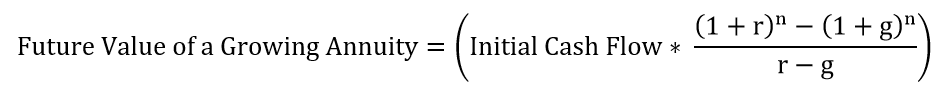#### This video shows how to calculate the future value of a growing annuity. Here is an example of a growing annuity: let’s say you decide to deposit \$100 in a savings account this year, \$110 in a savings account next year, and \$121 in a savings account the year after that. Each year there is a cash flow, but the amount of the cash flow is higher each year. The video provides a formula that shows you how to calculate the future value of a growing annuity, assuming that you know the initial cash flow, the growth rate of the cash flows, the number of periods, and the discount rate.

When we calculate the future value of a growing annuity, we are figuring out the ending balance of a series of cash flows that increase each period.  Let’s say you deposit \$100 in a savings account this year, \$110 in a savings account next year, and \$121 in a savings account the year after that.  You expect to have a return of 12%, and you’d like to know how much money you’ll have in the account after 3 years.  This is the future value of a growing annuity (the amount of the cash flow is growing by 10% each period) and it can be calculated using the formula below:where:

r = the discount rate

g = the growth rate (the rate by which the periodic cash flows increase each period)

n = the number of periods

Initial Cash Flow = the amount of the first cash flow

Plugging in the numbers from the example given earlier yields:Calculating the numbers above results in a figure of \$369.64.  Thus, if you make a series of deposits starting at \$100 and increasing by 10% each period, and you earn a return of 12% on your investment, you will have \$369.64 in your account at the end of 3 years.  That could buy you a lot of ice cream.